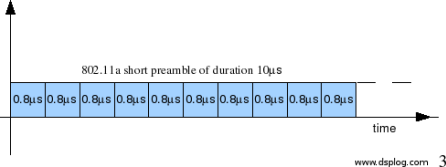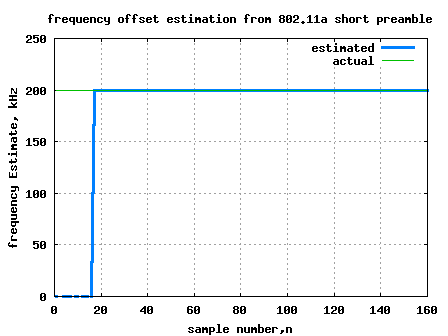- DSP log - http://www.dsplog.com -

Frequency offset estimation using 802.11a short preamble

Posted By Krishna Sankar On March 3, 2008 @ 6:11 am In OFDM | 83 Comments

From the previous post on OFDM (here ), we have understood that an OFDM waveform is made of sum of multiple sinusoidals (also called subcarriers) each modulated independently. In this post, let us try to understand the estimation of frequency offset in a typical OFDM receiver (using the short preamble specified per IEEE 802.11a specification  as a reference).

## Understanding frequency offset

In a typical wireless communication system, the signal to be transmitted is upconverted to a carrier frequency prior to transmission. The receiver is expected to tune to the same carrier frequency for downconverting the signal to baseband, prior to demodulation.

Figure: Up/down conversion

However, due to device impairments the carrier frequency of the receiver need not be same as the carrier frequency of the transmitter. When this happens, the received baseband signal, instead of being centered at DC (0MHz), will be centered at a frequency , where
.

The baseband representation is (ignoring noise),

, where

is the transmitted signal and

is the frequency offset.

## Frequency offset estimation in 802.11a OFDM preamble

From the IEEE 802.11a specifications  (Sec 17.3.3), it can be observed that each OFDM packet has a preamble structure formed using 10 short preambles of duration each. This short preamble is constructed by defining 12 subcarriers only (out of the available 52 subcarriers) where the modulation of individual subcarriers ensure a low peak to average power ratio .Figure: OFDM short preamble 802.11a specification

From the equation defined in the previous section,

.

Given that short preamble is perodic with ,

.

At the receiver as both and are known,

.

Taking angle() of both sides of the equation

.

So, the frequency offset is,

.## Simulation model

Simple Matlab/Octave script simulating generation of 802.11a short preamble, introducing frequency offset of 200kHz and estimating frequency offset is provided. Click here to download .Figure: Plot of frequency offset estimate using 802.11a short preamble

As can be observed starting from sample number 17 (i.e. after ) and till samples number 160 () the frequency offset estimate is available. For improving accuracy in the presence of noise, typically the output is accumulated prior to computation of angle.

## Maximum possible frequency offset which can be estimated

It is known that limits of the phase estimated by the angle() function is from . Plugging this to the above equation, the minimum value of frequency offset value which can be estimated is,

and the maximum value is,

.

From Sec17.3.9.4 (of IEEE 802.11a specification ), the center frequency tolerance is . With a carrier frequency of 5.8GHz, this specification corresponds to a frequency offset within the range . Thankfully, this is within the ‘estimatable’ range of frequency offsets which which can be estimated by the short preamble.

Note:
We have not discussed the effect of inter carrier interference (ICI) between the sub-carriers which happens in the presence of frequency offset. Maybe we can discuss in a later post.

## References

Hope this helps.
Krishna

URL to article: http://www.dsplog.com/2008/03/03/frequency-offset-estimation-using-80211a-short-preamble/

URLs in this post:

 here: http://www.dsplog.com/2008/02/03/understanding-an-ofdm-transmission/